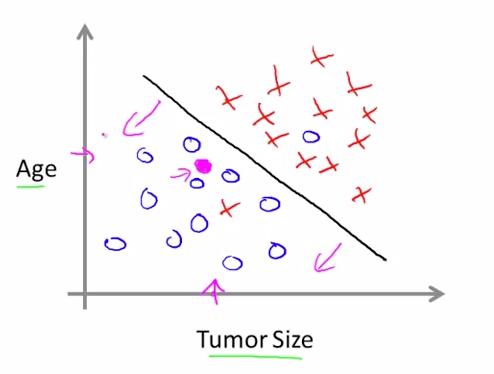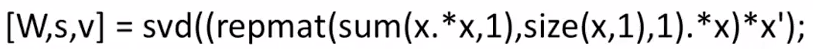WEEK1

The definition of machine learning

A computer program is said to learn from experience E with respect to some class of tasks T and performance measure P, if its performance at tasks in T, as measured by P, improves with experience E.

In most cases, the machine learning process is typed into two: Supervised learning and Unsupervised learning.

Supervisor learning

Giving the 'right answers' to the model learning.

Supervised learning problems are classified into two types of problems:

Regression problem and Classification problem.

Regression

Predict continuous output is obtained via single prediction function.

Classification

Discrete valued output is obtained via classification.Unsupervised learning

Learning without 'right answer'. This algorithm can be applied for example, clustering problem.

We do not need previous idea about what the label would be.

e.g. Cocktail party problem algorithmLinear regression problem

Suppose the date set is expressed with input x(i) and output y(i) , and the hypothesis $h$ is expressed as

$$h_\theta(x) = \theta_0 + \theta_1 x$$

the problem is converted into a problem to figure out a set of parameter $(\theta_0, \theta_1)$ so that this set is the best prediction accuracy. The prediction accuracy can be estimated with cost function $J(\theta_0, \theta_1)$ as

$$J(\theta_0, \theta_1) = \frac{1}{2m}\sum_{i=1}^{m}(\hat{y_i}-y_i)^2 = \frac{1}{2m}\sum_{i=1}^{m}(h_\theta (x_i)-y_i)^2$$

The cost function is called 'mean square error'. Now, the problem is as follows:

WEEK 11

Machine learning pipeline

Usually, the whole machine learning system is expressed as a pipeline of some machine learning steps.

e.g. Photo OCR system

Law picture -> Text area detection -> Character segmentation -> Character classification

Sliding windows

Sliding windows technique is used in some machine learning apllication, especially for photo detection problem.

To detect some components in a picture, you would configure a detection window, and then slide it along two axises.

i.e. If you want to analyze 200 * 200 pixel picture with 10 * 10, 15 * 15 and 20 * 20 size windows with 4 pixels per each "step", you would process it with about 750000 times.

Getting lots of data and artificail data

If you have not enough data to train your machine learning system, one way to obtain more positive data is to make artificial modification to original positive datasets. For example, rotating, distorting, stretching, and resizing. Linearly independent modifications are not efficient.

Adding purely random noise to your data usually doesn't help improveing training.

Ceiling analysis

If you have a machine learning pipeline and you want to improve the entire accuracy, but don't know where to be improved, the ceiling analysis is applicable. To each component, if its accuracy is 100%, how the entire accuracy becomes. If the value is large, that part is where you should put resource on.